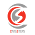# Column Design Calculation Excel File Free Download

### WSD and USD method Column Design Calculation

Comp Strength of Concrete, f'c = 4500 psi.
Yielding Strength of Steel, fy = 55000 psi.
Width of the Column, W = 16 in.
Steel ratio = 2%
Load, P = 300 kip.
For tied Rectangular Column, Ø = 0.65
For Spiral Column, Ø = 0.75

WSD ρg = 0.02
Clear Cover, CC = 2.5

Capacity Finder:
Area of the Column = 12 X 12
Area of steel for Rectangular Or Square Column = 7.2 in-squares
Radius for Circular Column = 5 in.
Area of steel for Circular Column, As = 1.86 in-squares.
Capacity of the Column:
Capacity of the Rectangular Column is = 478.015 Kip USD = 272.34 Kip WSD.
Capacity of the Circular  Column is = 218.57 Kip USD = 129.278 Kip WSD.

Calculation for Square Tied Column: (WSD)

1.Thanks for sharing knowledge of Column Design

1.2.Nice detail calculation of column Design, This article given me good knowledge of column design

1.3.Some people refer to a bifold window as “horizontal folding windows” or “horizontal bifold windows”.